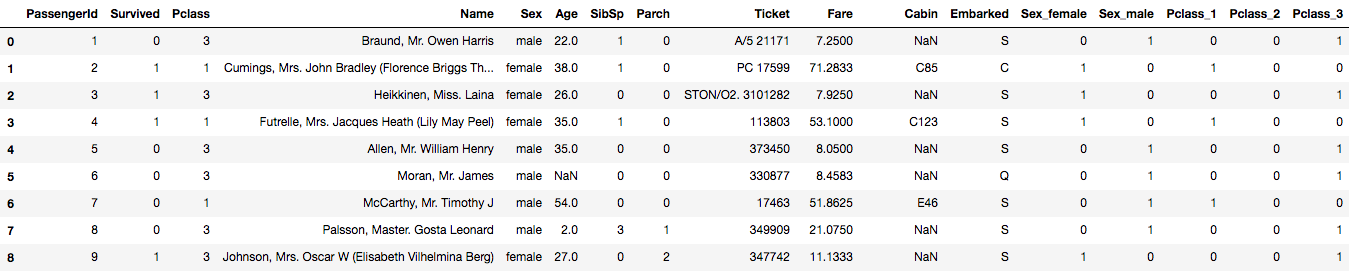As we previously mentioned, we will be looking into making our simplest classifier model better. One of the commonly used techniques used is categorical feature encoding. We will use one of the simpler and widely used types of encoders for demonstrating how it works and what it does.

One Hot Encoding - commonly used approach for encoding categorical data.

#### basic usage

We will build on our basic model from part 1, so head there in case you missed it.

Encoding categorical data into binary columns is easy with pandas - we can use the `pandas.get_dummies` method:

 ```1 2``` ```dummies = pd.get_dummies(df[column], prefix = column) df = pd.concat([df, dummies], axis = 1) ```

We create a dataframe of “dummies” - the categorical columns encoded into binary column with original column name as prefix - and merge it into our original dataframe.

To plug this into our simple script we can wrap these two lines in a function to prevent repeating code like so:

 ```1 2 3 4 5 6 7 8 9 10``` ```categorical_features = ["Sex", "Pclass"] def add_encoded_columns(df, column): dummies = pd.get_dummies(df[column], prefix = column) df = pd.concat([df, dummies], axis = 1) return df for feature in categorical_features: train = add_encoded_columns(train, feature) test = add_encoded_columns(test, feature) ```

This produces new columns:Next, when we choose columns for our model we replace the original “Sex” and “Pclass” columns with the newly created columns.

 `1` ```columns = ["Fare", "Sex_female", "Sex_male", "Pclass_1", "Pclass_2", "Pclass_3", "Age"] ```

Running the updated scripts produces a warning message:

 ```1 2 3 4 5 6 7 8``` ```python one-hot-encoding.py /usr/local/var/pyenv/versions/3.6.0/lib/python3.6/site-packages/sklearn/linear_model/logistic.py:758: ConvergenceWarning: lbfgs failed to converge. Increase the number of iterations. "of iterations.", ConvergenceWarning) Confusion matrix: [[93 17] [19 50]] Accuracy: 0.7988826815642458 ```

The warning specifically tells us how to fix the issue - increase the number of iterations: (We can see that the training set accuracy is a bit lower here, just below 80% vs the original 81% - a clear confirmation of the warning message)

 `1` ```classifier = LogisticRegression(random_state = 0, solver="lbfgs", max_iter = 200) ```

Doubling the maximum number of iterations does solve the converging issue, but does not improve on the score of our model.

#### the problem

Using One Hot Encoding does not improve the performance of our classifier, it actually lowers it by ~2%.

#### the reason

I am not an expert on the issue of variable encoding for ML algorithms, but there are different aspects that play their role:

1. Cardinality of data (e.g. 2 sexes vs 1000s of ZIP codes)
2. Model - linear regression and gradient boost will not take encoded values the same way, some models benefit from encoding, some do not.
3. Encoding type - numeric, one hot, binary, ordinal…there are many approaches when it comes to encoding categorical features.

#### what next

There are many great resources on the topic of encoding, I recommend reading about the topic a bit and then experimenting with different data sets and encoding types. I read these when I encountered lower performance after one-hot-encoding the Titanic data:

Keep learning!